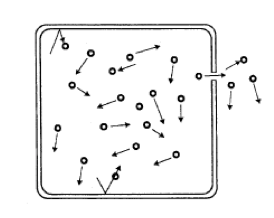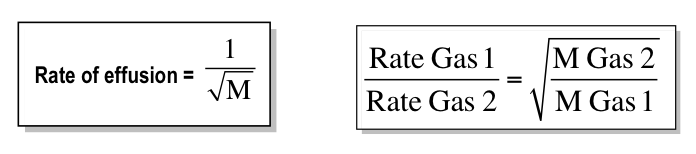Ch.1 - Intro to General Chemistry 2hrs & 53mins 0% complete Worksheet Ch.2 - Atoms & Elements 2hrs & 49mins 0% complete Worksheet Ch.3 - Chemical Reactions 3hrs & 25mins 0% complete Worksheet BONUS: Lab Techniques and Procedures 1hr & 38mins 0% complete Worksheet BONUS: Mathematical Operations and Functions 47mins 0% complete Worksheet Ch.4 - Chemical Quantities & Aqueous Reactions 3hrs & 30mins 0% complete Worksheet Ch.5 - Gases 3hrs & 47mins 0% complete Worksheet Ch.6 - Thermochemistry 2hrs & 28mins 0% complete Worksheet Ch.7 - Quantum Mechanics 2hrs & 35mins 0% complete Worksheet Ch.8 - Periodic Properties of the Elements 1hr & 57mins 0% complete Worksheet Ch.9 - Bonding & Molecular Structure 2hrs & 5mins 0% complete Worksheet Ch.10 - Molecular Shapes & Valence Bond Theory 1hr & 31mins 0% complete Worksheet Ch.11 - Liquids, Solids & Intermolecular Forces 3hrs & 40mins 0% complete Worksheet Ch.12 - Solutions 2hrs & 17mins 0% complete Worksheet Ch.13 - Chemical Kinetics 2hrs & 22mins 0% complete Worksheet Ch.14 - Chemical Equilibrium 2hrs & 26mins 0% complete Worksheet Ch.15 - Acid and Base Equilibrium 4hrs & 42mins 0% complete Worksheet Ch.16 - Aqueous Equilibrium 3hrs & 48mins 0% complete Worksheet Ch. 17 - Chemical Thermodynamics 1hr & 44mins 0% complete Worksheet Ch.18 - Electrochemistry 2hrs & 58mins 0% complete Worksheet Ch.19 - Nuclear Chemistry 1hr & 33mins 0% complete Worksheet Ch.20 - Organic Chemistry 3hrs 0% complete Worksheet Ch.22 - Chemistry of the Nonmetals 2hrs & 1min 0% complete Worksheet Ch.23 - Transition Metals and Coordination Compounds 1hr & 54mins 0% complete Worksheet

# Effusion

See all sections

Graham's Law states that the rate of effusion of a gas is inversely proportional to the square root of its molar mass.

###### Graham's Law of Effusion

Effusion involves a gas escaping a container through an opening.Concept #1: Understanding Graham's Law of Effusion.

Transcript

Welcome back guys. In this new video, we'll get to look at the effusion rates of different gases.
Tied with effusion is basically the concept of velocity and speed. Remember we use root mean square equations when we're dealing with the speed of one gas. We use effusion when we're comparing the speeds or velocities of multiple gases. That's when we use this concept.
Here we're going to say Graham's Law states that the rate of effusion of a gas is inversely proportional to the square root of its molar mass. What this is really saying is that the speed or rate at which a gas moves, so effusion is just seen as speed, the speed of a gas is the opposite of its weight.
What that means is if my speed is high, it's because the mass of my gas is low. Basically, the less you weigh as a gas, the faster you move. That's what effusion is trying to explain. Effusion looks and compares multiple gases to each other. Gas A moves faster than Gas B. Why is that? Well, Gas A must weigh less. That's why it's moving faster.
We're going to say when we're comparing the rates of two gases, we use this form of Graham's Law of Effusion. Now pay close attention. Basically, we're going to say the rate of Gas One is equal to its rate divided by the rate of Gas Two. We're going to say that equals the square root – look if Gas One's rate is on the top, then it's mass is on the bottom because remember Graham's Law says that rate and mass are inversely proportional. They're on opposite levels. Here if the rate of Gas Two is on the bottom, then its mass is on the top. We're going to have to take this approach to answer some of these questions.

When comparing the rate or speed of two gases then we must use Graham’s Law of EffusionExample #1: Calculate the ratio of the effusion rates of helium and methane (CH4).

The gas named first will represent gas 1 and the second gas will represent gas 2. Once that is established we simply use Graham’s Law of Effusion.

Example #2: Rank the following in order of increasing rate of effusion:

O2                    AlF5                  CO2                 Xe